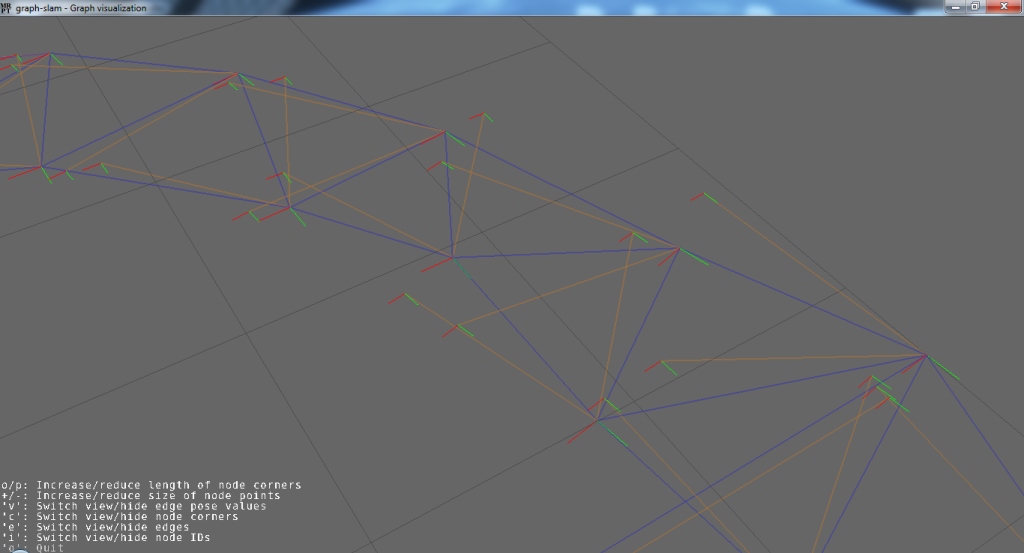Graph-SLAM maps

1. Text .graph file format

This file format was (to the best of my knowledge) first used in public software with TORO, and since then has been employed by other libraries and programs published in OpenSLAM.org.

Graphs in both 2D (x,y,phi) or 3D (x,y,z,yaw,pitch,roll) are supported. Files consist of plain text which is interpreted line by line. The first word in each line determines the type of that entry.
The following entry types exist:

• VERTEX2: A 2D node
id is a unique identifier for each node. phi is in radians.
• VERTEX3: A 3D node
id is a unique identifier for each node. yaw,pitch and roll are in radians.
• EDGE2: A 2D pose constraint
• EDGE3: A 3D pose constraint
• EQUIV: A “topological loop closure”, forcing two nodes to actually become the same.
• FIX: Defines “fixed” keyframes, that works as “anchors” to help the optimization problem to have one single solution. Fixes poses do not change during optimization.

2. Binary .graphbin file format

This format may be used if I/O disk performance is an issue. It consists of direct binary serializations of the corresponding objects, that is:

3. Related C++ code

Libraries:

•  mrpt-graphs contains abstract graph datatypes.
•  mrpt-graphslam contains graph-slam algorithms (Levenberg-Marquardt graph of constrainst optimization, etc.).

4. Example graph maps

The following graph-SLAM maps have been rendered with the C++ classes mentioned above, and the .graph files are those published wih TORO on OpenSLAM.org:

This view shows how the graph-slam program (by means functions in the namespace mrpt::opengl::graph_tools ) can show the mismatches between the pose of nodes and the constraints in the edges: